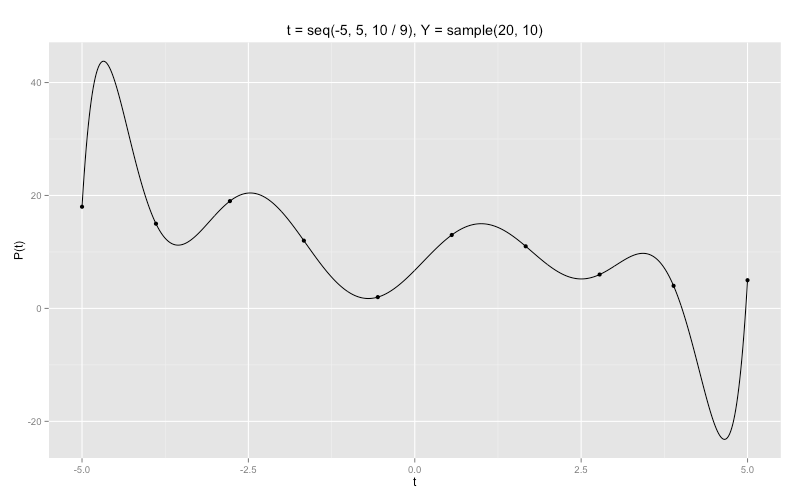Want to share your content on R-bloggers? click here if you have a blog, or here if you don't.

Let’s take $n$ distinct points on the real line:

Yay. We can now define the Lagrange Polynomials :

Why am I making you look at this beauté? Turns out there’s some neat mathematical properties - namely in the subject of polynomial interpolation. That’s a fancy way of saying ‘fit a polynomial through certain points’ but we’ll get to that.

Now, it’s easy to see that

since for $k = j$ every term in the product will be 1 ($t = t_k$ in the product), while for $k \ne j$ there will be one term with $t_j - t_j$ in the numerator (rendering the whole product to be 0). Keep in mind that the product above is not defined for the term where $j = k$ otherwise we’d have a divison-by-zero term.

See where this is going? Let’s introduce $y_1, …, y_n$ as arbitrary real numbers. How would we construct a polynomial that goes through the $(t_i, y_i)$ points? That is, can we find a polynomial $p$ such that $p(t_j) = y_j$ for each index $j = 1, …, n$? Hint: I didn’t write all that stuff up there for no reason.

Given what we know about $p_k(t_j)$ we can do the following:

Now we’ve defined an equation based on arbitrary $t_i$ and $y_i$ that will go through the points $(t_i, y_i)$ and behave otherwise elsewhere (we will see soon).

How do we code something like this up? As usual there’s a lot of different ways but I decided to go with closures as function factories because I tend to think functionally, and also because the code is a bit more concise in this case. First we can define a $p_k(t)$ function factory.

Given inputs k and ts (vector), we get back a $p_k$ that can be evaluated at any real point. It also has the above mentioned properties.

Next a $p(t)$ function factory.

It takes in a Y vector and a list of polynomials ($p_k(t)$) and outputs a real-valued function of $t$. The list of polynomials in this case is a list of functions (output of the p_k function).

Finally a quick function to create the combined polynomial given a vector of Y and T (constructing the $p_k$ polynomials in the process).

We see that it has the desired effect:

Finally, a few applications:We let t be 10 equispaced points between -1 and 1, and Y be the cube of those points. As we can see, the polynomial interpolation of those points looks to be roughly equal to $f(x) = x ^ 3$ which is pretty ‘good’.Now we try it with points that aren’t monotone increasing in both $t$ and $y$. The result is a little weird with sharp corners near the edges.

And what if we tried to interpolate on completely random points (i.e. not trying to ‘retrieve’ some sort of function transformation)?Pretty cool. You can play around with this but the polynomials have massive spikes near $t_1$ and $t_n$ if you introduce too many more points.

If you’re a statistician, alarms should be going off in your head as far as using polynomial interpolation to describe a generalized relationship between two vectors of data (overfitting anybody?). Any form of function fitting (even non-parametric) requires some pretty strong assumptions on the the smoothness of the curve involved. It also quickly becomes clear that if you add in three or more non-colinear points that the polynomial interpolation will start to have very sharp edges. This is where other options (i.e. linear regression) come into play and help fix the bias-variance problem we are observing. Also, more modern ‘smoothing’ techniques such as splining exist.

That being said, under the right conditions (monotonicity, non-complicated transformations, etc.) the polynomial retrieved from this method may not be a bad generalization of the relationship between $t$ and $y$. All in all, there are some cool mathematical properties at play here and this isn’t too shabby for something that was intuitively derived in the 1700s.